# 第11章

## 11.1 一些例子

``````#!bash
void f (int a)
{
switch (a)
{
case 0: printf ("zero
"); break;
case 1: printf ("one
"); break;
case 2: printf ("two
"); break;
default: printf ("something unknown
"); break;
};
};
``````

### 11.1.1 X86

``````#!bash
tv64 = -4       ; size = 4
_a\$ = 8         ; size = 4
_f  PROC
push    ebp
mov     ebp, esp
push    ecx
mov     eax, DWORD PTR _a\$[ebp]
mov     DWORD PTR tv64[ebp], eax
cmp     DWORD PTR tv64[ebp], 0
je      SHORT [email protected]/* <![CDATA[ */!function(t,e,r,n,c,a,p){try{t=document.currentScript||function(){for(t=document.getElementsByTagName('script'),e=t.length;e--;)if(t[e].getAttribute('data-cfhash'))return t[e]}();if(t&#038;&#038;(c=t.previousSibling)){p=t.parentNode;if(a=c.getAttribute('data-cfemail')){for(e='',r='0x'+a.substr(0,2)|0,n=2;a.length-n;n+=2)e+='%'+('0'+('0x'+a.substr(n,2)^r).toString(16)).slice(-2);p.replaceChild(document.createTextNode(decodeURIComponent(e)),c)}p.removeChild(t)}}catch(u){}}()/* ]]&gt; */
cmp     DWORD PTR tv64[ebp], 1
je      SHORT [email protected]/* <![CDATA[ */!function(t,e,r,n,c,a,p){try{t=document.currentScript||function(){for(t=document.getElementsByTagName('script'),e=t.length;e--;)if(t[e].getAttribute('data-cfhash'))return t[e]}();if(t&#038;&#038;(c=t.previousSibling)){p=t.parentNode;if(a=c.getAttribute('data-cfemail')){for(e='',r='0x'+a.substr(0,2)|0,n=2;a.length-n;n+=2)e+='%'+('0'+('0x'+a.substr(n,2)^r).toString(16)).slice(-2);p.replaceChild(document.createTextNode(decodeURIComponent(e)),c)}p.removeChild(t)}}catch(u){}}()/* ]]&gt; */
cmp     DWORD PTR tv64[ebp], 2
je      SHORT [email protected]/* <![CDATA[ */!function(t,e,r,n,c,a,p){try{t=document.currentScript||function(){for(t=document.getElementsByTagName('script'),e=t.length;e--;)if(t[e].getAttribute('data-cfhash'))return t[e]}();if(t&#038;&#038;(c=t.previousSibling)){p=t.parentNode;if(a=c.getAttribute('data-cfemail')){for(e='',r='0x'+a.substr(0,2)|0,n=2;a.length-n;n+=2)e+='%'+('0'+('0x'+a.substr(n,2)^r).toString(16)).slice(-2);p.replaceChild(document.createTextNode(decodeURIComponent(e)),c)}p.removeChild(t)}}catch(u){}}()/* ]]&gt; */
jmp     SHORT [email protected]/* <![CDATA[ */!function(t,e,r,n,c,a,p){try{t=document.currentScript||function(){for(t=document.getElementsByTagName('script'),e=t.length;e--;)if(t[e].getAttribute('data-cfhash'))return t[e]}();if(t&#038;&#038;(c=t.previousSibling)){p=t.parentNode;if(a=c.getAttribute('data-cfemail')){for(e='',r='0x'+a.substr(0,2)|0,n=2;a.length-n;n+=2)e+='%'+('0'+('0x'+a.substr(n,2)^r).toString(16)).slice(-2);p.replaceChild(document.createTextNode(decodeURIComponent(e)),c)}p.removeChild(t)}}catch(u){}}()/* ]]&gt; */
[email protected]/* <![CDATA[ */!function(t,e,r,n,c,a,p){try{t=document.currentScript||function(){for(t=document.getElementsByTagName('script'),e=t.length;e--;)if(t[e].getAttribute('data-cfhash'))return t[e]}();if(t&#038;&#038;(c=t.previousSibling)){p=t.parentNode;if(a=c.getAttribute('data-cfemail')){for(e='',r='0x'+a.substr(0,2)|0,n=2;a.length-n;n+=2)e+='%'+('0'+('0x'+a.substr(n,2)^r).toString(16)).slice(-2);p.replaceChild(document.createTextNode(decodeURIComponent(e)),c)}p.removeChild(t)}}catch(u){}}()/* ]]&gt; */:
push    OFFSET \$SG739 ; ’zero’, 0aH, 00H
call    _printf
jmp     SHORT [email protected]/* <![CDATA[ */!function(t,e,r,n,c,a,p){try{t=document.currentScript||function(){for(t=document.getElementsByTagName('script'),e=t.length;e--;)if(t[e].getAttribute('data-cfhash'))return t[e]}();if(t&#038;&#038;(c=t.previousSibling)){p=t.parentNode;if(a=c.getAttribute('data-cfemail')){for(e='',r='0x'+a.substr(0,2)|0,n=2;a.length-n;n+=2)e+='%'+('0'+('0x'+a.substr(n,2)^r).toString(16)).slice(-2);p.replaceChild(document.createTextNode(decodeURIComponent(e)),c)}p.removeChild(t)}}catch(u){}}()/* ]]&gt; */
[email protected]/* <![CDATA[ */!function(t,e,r,n,c,a,p){try{t=document.currentScript||function(){for(t=document.getElementsByTagName('script'),e=t.length;e--;)if(t[e].getAttribute('data-cfhash'))return t[e]}();if(t&#038;&#038;(c=t.previousSibling)){p=t.parentNode;if(a=c.getAttribute('data-cfemail')){for(e='',r='0x'+a.substr(0,2)|0,n=2;a.length-n;n+=2)e+='%'+('0'+('0x'+a.substr(n,2)^r).toString(16)).slice(-2);p.replaceChild(document.createTextNode(decodeURIComponent(e)),c)}p.removeChild(t)}}catch(u){}}()/* ]]&gt; */:
push    OFFSET \$SG741 ; ’one’, 0aH, 00H
call    _printf
jmp     SHORT [email protected]/* <![CDATA[ */!function(t,e,r,n,c,a,p){try{t=document.currentScript||function(){for(t=document.getElementsByTagName('script'),e=t.length;e--;)if(t[e].getAttribute('data-cfhash'))return t[e]}();if(t&#038;&#038;(c=t.previousSibling)){p=t.parentNode;if(a=c.getAttribute('data-cfemail')){for(e='',r='0x'+a.substr(0,2)|0,n=2;a.length-n;n+=2)e+='%'+('0'+('0x'+a.substr(n,2)^r).toString(16)).slice(-2);p.replaceChild(document.createTextNode(decodeURIComponent(e)),c)}p.removeChild(t)}}catch(u){}}()/* ]]&gt; */
[email protected]/* <![CDATA[ */!function(t,e,r,n,c,a,p){try{t=document.currentScript||function(){for(t=document.getElementsByTagName('script'),e=t.length;e--;)if(t[e].getAttribute('data-cfhash'))return t[e]}();if(t&#038;&#038;(c=t.previousSibling)){p=t.parentNode;if(a=c.getAttribute('data-cfemail')){for(e='',r='0x'+a.substr(0,2)|0,n=2;a.length-n;n+=2)e+='%'+('0'+('0x'+a.substr(n,2)^r).toString(16)).slice(-2);p.replaceChild(document.createTextNode(decodeURIComponent(e)),c)}p.removeChild(t)}}catch(u){}}()/* ]]&gt; */:
push    OFFSET \$SG743 ; ’two’, 0aH, 00H
call    _printf
jmp     SHORT [email protected]/* <![CDATA[ */!function(t,e,r,n,c,a,p){try{t=document.currentScript||function(){for(t=document.getElementsByTagName('script'),e=t.length;e--;)if(t[e].getAttribute('data-cfhash'))return t[e]}();if(t&#038;&#038;(c=t.previousSibling)){p=t.parentNode;if(a=c.getAttribute('data-cfemail')){for(e='',r='0x'+a.substr(0,2)|0,n=2;a.length-n;n+=2)e+='%'+('0'+('0x'+a.substr(n,2)^r).toString(16)).slice(-2);p.replaceChild(document.createTextNode(decodeURIComponent(e)),c)}p.removeChild(t)}}catch(u){}}()/* ]]&gt; */
[email protected]/* <![CDATA[ */!function(t,e,r,n,c,a,p){try{t=document.currentScript||function(){for(t=document.getElementsByTagName('script'),e=t.length;e--;)if(t[e].getAttribute('data-cfhash'))return t[e]}();if(t&#038;&#038;(c=t.previousSibling)){p=t.parentNode;if(a=c.getAttribute('data-cfemail')){for(e='',r='0x'+a.substr(0,2)|0,n=2;a.length-n;n+=2)e+='%'+('0'+('0x'+a.substr(n,2)^r).toString(16)).slice(-2);p.replaceChild(document.createTextNode(decodeURIComponent(e)),c)}p.removeChild(t)}}catch(u){}}()/* ]]&gt; */:
push    OFFSET \$SG745 ; ’something unknown’, 0aH, 00H
call    _printf
[email protected]/* <![CDATA[ */!function(t,e,r,n,c,a,p){try{t=document.currentScript||function(){for(t=document.getElementsByTagName('script'),e=t.length;e--;)if(t[e].getAttribute('data-cfhash'))return t[e]}();if(t&#038;&#038;(c=t.previousSibling)){p=t.parentNode;if(a=c.getAttribute('data-cfemail')){for(e='',r='0x'+a.substr(0,2)|0,n=2;a.length-n;n+=2)e+='%'+('0'+('0x'+a.substr(n,2)^r).toString(16)).slice(-2);p.replaceChild(document.createTextNode(decodeURIComponent(e)),c)}p.removeChild(t)}}catch(u){}}()/* ]]&gt; */:
mov     esp, ebp
pop     ebp
ret     0
_f    ENDP
``````

``````#!cpp
void f (int a)
{
if (a==0)
printf ("zero
");
else if (a==1)
printf ("one
");
else if (a==2)
printf ("two
");
else
printf ("something unknown
");
};
``````

``````#!bash
_a\$ = 8                 ; size = 4
_f  PROC
mov     eax, DWORD PTR _a\$[esp-4]
sub     eax, 0
je      SHORT [email protected]/* <![CDATA[ */!function(t,e,r,n,c,a,p){try{t=document.currentScript||function(){for(t=document.getElementsByTagName('script'),e=t.length;e--;)if(t[e].getAttribute('data-cfhash'))return t[e]}();if(t&#038;&#038;(c=t.previousSibling)){p=t.parentNode;if(a=c.getAttribute('data-cfemail')){for(e='',r='0x'+a.substr(0,2)|0,n=2;a.length-n;n+=2)e+='%'+('0'+('0x'+a.substr(n,2)^r).toString(16)).slice(-2);p.replaceChild(document.createTextNode(decodeURIComponent(e)),c)}p.removeChild(t)}}catch(u){}}()/* ]]&gt; */
sub     eax, 1
je      SHORT [email protected]/* <![CDATA[ */!function(t,e,r,n,c,a,p){try{t=document.currentScript||function(){for(t=document.getElementsByTagName('script'),e=t.length;e--;)if(t[e].getAttribute('data-cfhash'))return t[e]}();if(t&#038;&#038;(c=t.previousSibling)){p=t.parentNode;if(a=c.getAttribute('data-cfemail')){for(e='',r='0x'+a.substr(0,2)|0,n=2;a.length-n;n+=2)e+='%'+('0'+('0x'+a.substr(n,2)^r).toString(16)).slice(-2);p.replaceChild(document.createTextNode(decodeURIComponent(e)),c)}p.removeChild(t)}}catch(u){}}()/* ]]&gt; */
sub     eax, 1
je      SHORT [email protected]/* <![CDATA[ */!function(t,e,r,n,c,a,p){try{t=document.currentScript||function(){for(t=document.getElementsByTagName('script'),e=t.length;e--;)if(t[e].getAttribute('data-cfhash'))return t[e]}();if(t&#038;&#038;(c=t.previousSibling)){p=t.parentNode;if(a=c.getAttribute('data-cfemail')){for(e='',r='0x'+a.substr(0,2)|0,n=2;a.length-n;n+=2)e+='%'+('0'+('0x'+a.substr(n,2)^r).toString(16)).slice(-2);p.replaceChild(document.createTextNode(decodeURIComponent(e)),c)}p.removeChild(t)}}catch(u){}}()/* ]]&gt; */
mov     DWORD PTR _a\$[esp-4], OFFSET \$SG791 ; ’something unknown’, 0aH, 00H
jmp     _printf
[email protected]/* <![CDATA[ */!function(t,e,r,n,c,a,p){try{t=document.currentScript||function(){for(t=document.getElementsByTagName('script'),e=t.length;e--;)if(t[e].getAttribute('data-cfhash'))return t[e]}();if(t&#038;&#038;(c=t.previousSibling)){p=t.parentNode;if(a=c.getAttribute('data-cfemail')){for(e='',r='0x'+a.substr(0,2)|0,n=2;a.length-n;n+=2)e+='%'+('0'+('0x'+a.substr(n,2)^r).toString(16)).slice(-2);p.replaceChild(document.createTextNode(decodeURIComponent(e)),c)}p.removeChild(t)}}catch(u){}}()/* ]]&gt; */:
mov     DWORD PTR _a\$[esp-4], OFFSET \$SG789 ; ’two’, 0aH, 00H
jmp     _printf
[email protected]/* <![CDATA[ */!function(t,e,r,n,c,a,p){try{t=document.currentScript||function(){for(t=document.getElementsByTagName('script'),e=t.length;e--;)if(t[e].getAttribute('data-cfhash'))return t[e]}();if(t&#038;&#038;(c=t.previousSibling)){p=t.parentNode;if(a=c.getAttribute('data-cfemail')){for(e='',r='0x'+a.substr(0,2)|0,n=2;a.length-n;n+=2)e+='%'+('0'+('0x'+a.substr(n,2)^r).toString(16)).slice(-2);p.replaceChild(document.createTextNode(decodeURIComponent(e)),c)}p.removeChild(t)}}catch(u){}}()/* ]]&gt; */:
mov     DWORD PTR _a\$[esp-4], OFFSET \$SG787 ; ’one’, 0aH, 00H
jmp     _printf
[email protected]/* <![CDATA[ */!function(t,e,r,n,c,a,p){try{t=document.currentScript||function(){for(t=document.getElementsByTagName('script'),e=t.length;e--;)if(t[e].getAttribute('data-cfhash'))return t[e]}();if(t&#038;&#038;(c=t.previousSibling)){p=t.parentNode;if(a=c.getAttribute('data-cfemail')){for(e='',r='0x'+a.substr(0,2)|0,n=2;a.length-n;n+=2)e+='%'+('0'+('0x'+a.substr(n,2)^r).toString(16)).slice(-2);p.replaceChild(document.createTextNode(decodeURIComponent(e)),c)}p.removeChild(t)}}catch(u){}}()/* ]]&gt; */:
mov     DWORD PTR _a\$[esp-4], OFFSET \$SG785 ; ’zero’, 0aH, 00H
jmp     _printf
_f ENDP
``````

（译注：SUB操作会重置零标志位ZF，但是MOV不会设置标志位，而JE将只有在ZF标志位设置之后才会跳转。如果需要基于EAX的值来做JE跳转的话，是需要用这个方法设置标志位的）。

``````· ESP —— 指向返回地址
· ESP+4 —— 指向变量a （也即参数）
``````

ARM编译器也有类似的优化，请见5.3.2节“带有多个参数的printf()函数调用”。

### 11.1.2 ARM： 优化后的 Keil + ARM 模式

``````#!bash
.text:0000014C             f1
.text:0000014C 00 00 50 E3          CMP R0, #0
.text:00000150 13 0E 8F 02          ADREQ R0, aZero     ; "zero
"
.text:00000154 05 00 00 0A          BEQ loc_170
.text:00000158 01 00 50 E3          CMP R0, #1
.text:0000015C 4B 0F 8F 02          ADREQ R0, aOne      ; "one
"
.text:00000160 02 00 00 0A          BEQ loc_170
.text:00000164 02 00 50 E3          CMP R0, #2
.text:00000168 4A 0F 8F 12          ADRNE R0, aSomethingUnkno ; "something unknown
"
.text:0000016C 4E 0F 8F 02          ADREQ R0, aTwo      ; "two
"
.text:00000170
.text:00000170                      loc_170             ; CODE XREF: f1+8
.text:00000170                                          ; f1+14
.text:00000170 78 18 00 EA          B __2printf
``````

”到R0中，因为a在之前已经和0、1做过是否相等的判断了，这里我们可以假定a并不等于0或者1。并且，如果R0=2，a指向的字符串“two

### 11.1.3 ARM： 优化后的 Keil + thumb 模式

``````#!bash
.text:000000D4          f1
.text:000000D4 10 B5            PUSH    {R4,LR}
.text:000000D6 00 28            CMP     R0, #0
.text:000000D8 05 D0            BEQ     zero_case
.text:000000DA 01 28            CMP     R0, #1
.text:000000DC 05 D0            BEQ     one_case
.text:000000DE 02 28            CMP     R0, #2
.text:000000E0 05 D0            BEQ     two_case
.text:000000E2 91 A0            ADR     R0, aSomethingUnkno ; "something unknown
"
.text:000000E4 04 E0            B       default_case
.text:000000E6 ;
-------------------------------------------------------------------------
.text:000000E6          zero_case                           ; CODE XREF: f1+4
.text:000000E6 95 A0            ADR     R0, aZero           ; "zero
"
.text:000000E8 02 E0            B       default_case
.text:000000EA ;
-------------------------------------------------------------------------
.text:000000EA          one_case                            ; CODE XREF: f1+8
.text:000000EA 96 A0            ADR     R0, aOne            ; "one
"
.text:000000EC 00 E0            B       default_case
.text:000000EE          ;
-------------------------------------------------------------------------
.text:000000EE          two_case                            ; CODE XREF: f1+C
.text:000000EE 97 A0            ADR     R0, aTwo            ; "two
"
.text:000000F0                  default_case                ; CODE XREF: f1+10
.text:000000F0                                              ; f1+14
.text:000000F0 06 F0 7E F8      BL      __2printf
.text:000000F4 10 BD            POP     {R4,PC}
.text:000000F4           ; End of function f1
``````

## 11.2 许多例子

``````#!cpp
void f (int a)
{
switch (a)
{
case 0: printf ("zero
"); break;
case 1: printf ("one
"); break;
case 2: printf ("two
"); break;
case 3: printf ("three
"); break;
case 4: printf ("four
"); break;
default: printf ("something unknown
"); break;
};
};
``````

### 11.2.1 x86

``````#!bash
tv64 = -4           ; size = 4
_a\$ = 8             ; size = 4
_f      PROC
push    ebp
mov     ebp, esp
push    ecx
mov     eax, DWORD PTR _a\$[ebp]
mov     DWORD PTR tv64[ebp], eax
cmp     DWORD PTR tv64[ebp], 4
ja      SHORT [email protected]/* <![CDATA[ */!function(t,e,r,n,c,a,p){try{t=document.currentScript||function(){for(t=document.getElementsByTagName('script'),e=t.length;e--;)if(t[e].getAttribute('data-cfhash'))return t[e]}();if(t&#038;&#038;(c=t.previousSibling)){p=t.parentNode;if(a=c.getAttribute('data-cfemail')){for(e='',r='0x'+a.substr(0,2)|0,n=2;a.length-n;n+=2)e+='%'+('0'+('0x'+a.substr(n,2)^r).toString(16)).slice(-2);p.replaceChild(document.createTextNode(decodeURIComponent(e)),c)}p.removeChild(t)}}catch(u){}}()/* ]]&gt; */
mov     ecx, DWORD PTR tv64[ebp]
jmp     DWORD PTR [email protected]/* <![CDATA[ */!function(t,e,r,n,c,a,p){try{t=document.currentScript||function(){for(t=document.getElementsByTagName('script'),e=t.length;e--;)if(t[e].getAttribute('data-cfhash'))return t[e]}();if(t&#038;&#038;(c=t.previousSibling)){p=t.parentNode;if(a=c.getAttribute('data-cfemail')){for(e='',r='0x'+a.substr(0,2)|0,n=2;a.length-n;n+=2)e+='%'+('0'+('0x'+a.substr(n,2)^r).toString(16)).slice(-2);p.replaceChild(document.createTextNode(decodeURIComponent(e)),c)}p.removeChild(t)}}catch(u){}}()/* ]]&gt; */[ecx*4]
[email protected]/* <![CDATA[ */!function(t,e,r,n,c,a,p){try{t=document.currentScript||function(){for(t=document.getElementsByTagName('script'),e=t.length;e--;)if(t[e].getAttribute('data-cfhash'))return t[e]}();if(t&#038;&#038;(c=t.previousSibling)){p=t.parentNode;if(a=c.getAttribute('data-cfemail')){for(e='',r='0x'+a.substr(0,2)|0,n=2;a.length-n;n+=2)e+='%'+('0'+('0x'+a.substr(n,2)^r).toString(16)).slice(-2);p.replaceChild(document.createTextNode(decodeURIComponent(e)),c)}p.removeChild(t)}}catch(u){}}()/* ]]&gt; */:
push    OFFSET \$SG739 ; ’zero’, 0aH, 00H
call    _printf
jmp     SHORT [email protected]/* <![CDATA[ */!function(t,e,r,n,c,a,p){try{t=document.currentScript||function(){for(t=document.getElementsByTagName('script'),e=t.length;e--;)if(t[e].getAttribute('data-cfhash'))return t[e]}();if(t&#038;&#038;(c=t.previousSibling)){p=t.parentNode;if(a=c.getAttribute('data-cfemail')){for(e='',r='0x'+a.substr(0,2)|0,n=2;a.length-n;n+=2)e+='%'+('0'+('0x'+a.substr(n,2)^r).toString(16)).slice(-2);p.replaceChild(document.createTextNode(decodeURIComponent(e)),c)}p.removeChild(t)}}catch(u){}}()/* ]]&gt; */
[email protected]/* <![CDATA[ */!function(t,e,r,n,c,a,p){try{t=document.currentScript||function(){for(t=document.getElementsByTagName('script'),e=t.length;e--;)if(t[e].getAttribute('data-cfhash'))return t[e]}();if(t&#038;&#038;(c=t.previousSibling)){p=t.parentNode;if(a=c.getAttribute('data-cfemail')){for(e='',r='0x'+a.substr(0,2)|0,n=2;a.length-n;n+=2)e+='%'+('0'+('0x'+a.substr(n,2)^r).toString(16)).slice(-2);p.replaceChild(document.createTextNode(decodeURIComponent(e)),c)}p.removeChild(t)}}catch(u){}}()/* ]]&gt; */:
push    OFFSET \$SG741 ; ’one’, 0aH, 00H
call    _printf
jmp     SHORT [email protected]/* <![CDATA[ */!function(t,e,r,n,c,a,p){try{t=document.currentScript||function(){for(t=document.getElementsByTagName('script'),e=t.length;e--;)if(t[e].getAttribute('data-cfhash'))return t[e]}();if(t&#038;&#038;(c=t.previousSibling)){p=t.parentNode;if(a=c.getAttribute('data-cfemail')){for(e='',r='0x'+a.substr(0,2)|0,n=2;a.length-n;n+=2)e+='%'+('0'+('0x'+a.substr(n,2)^r).toString(16)).slice(-2);p.replaceChild(document.createTextNode(decodeURIComponent(e)),c)}p.removeChild(t)}}catch(u){}}()/* ]]&gt; */
[email protected]/* <![CDATA[ */!function(t,e,r,n,c,a,p){try{t=document.currentScript||function(){for(t=document.getElementsByTagName('script'),e=t.length;e--;)if(t[e].getAttribute('data-cfhash'))return t[e]}();if(t&#038;&#038;(c=t.previousSibling)){p=t.parentNode;if(a=c.getAttribute('data-cfemail')){for(e='',r='0x'+a.substr(0,2)|0,n=2;a.length-n;n+=2)e+='%'+('0'+('0x'+a.substr(n,2)^r).toString(16)).slice(-2);p.replaceChild(document.createTextNode(decodeURIComponent(e)),c)}p.removeChild(t)}}catch(u){}}()/* ]]&gt; */:
push    OFFSET \$SG743 ; ’two’, 0aH, 00H
call    _printf
jmp     SHORT [email protected]/* <![CDATA[ */!function(t,e,r,n,c,a,p){try{t=document.currentScript||function(){for(t=document.getElementsByTagName('script'),e=t.length;e--;)if(t[e].getAttribute('data-cfhash'))return t[e]}();if(t&#038;&#038;(c=t.previousSibling)){p=t.parentNode;if(a=c.getAttribute('data-cfemail')){for(e='',r='0x'+a.substr(0,2)|0,n=2;a.length-n;n+=2)e+='%'+('0'+('0x'+a.substr(n,2)^r).toString(16)).slice(-2);p.replaceChild(document.createTextNode(decodeURIComponent(e)),c)}p.removeChild(t)}}catch(u){}}()/* ]]&gt; */
[email protected]/* <![CDATA[ */!function(t,e,r,n,c,a,p){try{t=document.currentScript||function(){for(t=document.getElementsByTagName('script'),e=t.length;e--;)if(t[e].getAttribute('data-cfhash'))return t[e]}();if(t&#038;&#038;(c=t.previousSibling)){p=t.parentNode;if(a=c.getAttribute('data-cfemail')){for(e='',r='0x'+a.substr(0,2)|0,n=2;a.length-n;n+=2)e+='%'+('0'+('0x'+a.substr(n,2)^r).toString(16)).slice(-2);p.replaceChild(document.createTextNode(decodeURIComponent(e)),c)}p.removeChild(t)}}catch(u){}}()/* ]]&gt; */:
push    OFFSET \$SG745 ; ’three’, 0aH, 00H
call    _printf
jmp     SHORT [email protected]/* <![CDATA[ */!function(t,e,r,n,c,a,p){try{t=document.currentScript||function(){for(t=document.getElementsByTagName('script'),e=t.length;e--;)if(t[e].getAttribute('data-cfhash'))return t[e]}();if(t&#038;&#038;(c=t.previousSibling)){p=t.parentNode;if(a=c.getAttribute('data-cfemail')){for(e='',r='0x'+a.substr(0,2)|0,n=2;a.length-n;n+=2)e+='%'+('0'+('0x'+a.substr(n,2)^r).toString(16)).slice(-2);p.replaceChild(document.createTextNode(decodeURIComponent(e)),c)}p.removeChild(t)}}catch(u){}}()/* ]]&gt; */
[email protected]/* <![CDATA[ */!function(t,e,r,n,c,a,p){try{t=document.currentScript||function(){for(t=document.getElementsByTagName('script'),e=t.length;e--;)if(t[e].getAttribute('data-cfhash'))return t[e]}();if(t&#038;&#038;(c=t.previousSibling)){p=t.parentNode;if(a=c.getAttribute('data-cfemail')){for(e='',r='0x'+a.substr(0,2)|0,n=2;a.length-n;n+=2)e+='%'+('0'+('0x'+a.substr(n,2)^r).toString(16)).slice(-2);p.replaceChild(document.createTextNode(decodeURIComponent(e)),c)}p.removeChild(t)}}catch(u){}}()/* ]]&gt; */:
push    OFFSET \$SG747 ; ’four’, 0aH, 00H
call    _printf
jmp     SHORT [email protected]/* <![CDATA[ */!function(t,e,r,n,c,a,p){try{t=document.currentScript||function(){for(t=document.getElementsByTagName('script'),e=t.length;e--;)if(t[e].getAttribute('data-cfhash'))return t[e]}();if(t&#038;&#038;(c=t.previousSibling)){p=t.parentNode;if(a=c.getAttribute('data-cfemail')){for(e='',r='0x'+a.substr(0,2)|0,n=2;a.length-n;n+=2)e+='%'+('0'+('0x'+a.substr(n,2)^r).toString(16)).slice(-2);p.replaceChild(document.createTextNode(decodeURIComponent(e)),c)}p.removeChild(t)}}catch(u){}}()/* ]]&gt; */
[email protected]/* <![CDATA[ */!function(t,e,r,n,c,a,p){try{t=document.currentScript||function(){for(t=document.getElementsByTagName('script'),e=t.length;e--;)if(t[e].getAttribute('data-cfhash'))return t[e]}();if(t&#038;&#038;(c=t.previousSibling)){p=t.parentNode;if(a=c.getAttribute('data-cfemail')){for(e='',r='0x'+a.substr(0,2)|0,n=2;a.length-n;n+=2)e+='%'+('0'+('0x'+a.substr(n,2)^r).toString(16)).slice(-2);p.replaceChild(document.createTextNode(decodeURIComponent(e)),c)}p.removeChild(t)}}catch(u){}}()/* ]]&gt; */:
push    OFFSET \$SG749 ; ’something unknown’, 0aH, 00H
call    _printf
[email protected]/* <![CDATA[ */!function(t,e,r,n,c,a,p){try{t=document.currentScript||function(){for(t=document.getElementsByTagName('script'),e=t.length;e--;)if(t[e].getAttribute('data-cfhash'))return t[e]}();if(t&#038;&#038;(c=t.previousSibling)){p=t.parentNode;if(a=c.getAttribute('data-cfemail')){for(e='',r='0x'+a.substr(0,2)|0,n=2;a.length-n;n+=2)e+='%'+('0'+('0x'+a.substr(n,2)^r).toString(16)).slice(-2);p.replaceChild(document.createTextNode(decodeURIComponent(e)),c)}p.removeChild(t)}}catch(u){}}()/* ]]&gt; */:
mov     esp, ebp
pop     ebp
ret     0
[email protected]/* <![CDATA[ */!function(t,e,r,n,c,a,p){try{t=document.currentScript||function(){for(t=document.getElementsByTagName('script'),e=t.length;e--;)if(t[e].getAttribute('data-cfhash'))return t[e]}();if(t&#038;&#038;(c=t.previousSibling)){p=t.parentNode;if(a=c.getAttribute('data-cfemail')){for(e='',r='0x'+a.substr(0,2)|0,n=2;a.length-n;n+=2)e+='%'+('0'+('0x'+a.substr(n,2)^r).toString(16)).slice(-2);p.replaceChild(document.createTextNode(decodeURIComponent(e)),c)}p.removeChild(t)}}catch(u){}}()/* ]]&gt; */:
DD  [email protected]/* <![CDATA[ */!function(t,e,r,n,c,a,p){try{t=document.currentScript||function(){for(t=document.getElementsByTagName('script'),e=t.length;e--;)if(t[e].getAttribute('data-cfhash'))return t[e]}();if(t&#038;&#038;(c=t.previousSibling)){p=t.parentNode;if(a=c.getAttribute('data-cfemail')){for(e='',r='0x'+a.substr(0,2)|0,n=2;a.length-n;n+=2)e+='%'+('0'+('0x'+a.substr(n,2)^r).toString(16)).slice(-2);p.replaceChild(document.createTextNode(decodeURIComponent(e)),c)}p.removeChild(t)}}catch(u){}}()/* ]]&gt; */ ; 0
DD  [email protected]/* <![CDATA[ */!function(t,e,r,n,c,a,p){try{t=document.currentScript||function(){for(t=document.getElementsByTagName('script'),e=t.length;e--;)if(t[e].getAttribute('data-cfhash'))return t[e]}();if(t&#038;&#038;(c=t.previousSibling)){p=t.parentNode;if(a=c.getAttribute('data-cfemail')){for(e='',r='0x'+a.substr(0,2)|0,n=2;a.length-n;n+=2)e+='%'+('0'+('0x'+a.substr(n,2)^r).toString(16)).slice(-2);p.replaceChild(document.createTextNode(decodeURIComponent(e)),c)}p.removeChild(t)}}catch(u){}}()/* ]]&gt; */ ; 1
DD  [email protected]/* <![CDATA[ */!function(t,e,r,n,c,a,p){try{t=document.currentScript||function(){for(t=document.getElementsByTagName('script'),e=t.length;e--;)if(t[e].getAttribute('data-cfhash'))return t[e]}();if(t&#038;&#038;(c=t.previousSibling)){p=t.parentNode;if(a=c.getAttribute('data-cfemail')){for(e='',r='0x'+a.substr(0,2)|0,n=2;a.length-n;n+=2)e+='%'+('0'+('0x'+a.substr(n,2)^r).toString(16)).slice(-2);p.replaceChild(document.createTextNode(decodeURIComponent(e)),c)}p.removeChild(t)}}catch(u){}}()/* ]]&gt; */ ; 2
DD  [email protected]/* <![CDATA[ */!function(t,e,r,n,c,a,p){try{t=document.currentScript||function(){for(t=document.getElementsByTagName('script'),e=t.length;e--;)if(t[e].getAttribute('data-cfhash'))return t[e]}();if(t&#038;&#038;(c=t.previousSibling)){p=t.parentNode;if(a=c.getAttribute('data-cfemail')){for(e='',r='0x'+a.substr(0,2)|0,n=2;a.length-n;n+=2)e+='%'+('0'+('0x'+a.substr(n,2)^r).toString(16)).slice(-2);p.replaceChild(document.createTextNode(decodeURIComponent(e)),c)}p.removeChild(t)}}catch(u){}}()/* ]]&gt; */ ; 3
DD  [email protected]/* <![CDATA[ */!function(t,e,r,n,c,a,p){try{t=document.currentScript||function(){for(t=document.getElementsByTagName('script'),e=t.length;e--;)if(t[e].getAttribute('data-cfhash'))return t[e]}();if(t&#038;&#038;(c=t.previousSibling)){p=t.parentNode;if(a=c.getAttribute('data-cfemail')){for(e='',r='0x'+a.substr(0,2)|0,n=2;a.length-n;n+=2)e+='%'+('0'+('0x'+a.substr(n,2)^r).toString(16)).slice(-2);p.replaceChild(document.createTextNode(decodeURIComponent(e)),c)}p.removeChild(t)}}catch(u){}}()/* ]]&gt; */ ; 4
_f     ENDP
``````

``````#!bash
public f
f       proc near ; CODE XREF: main+10

var_18  = dword ptr -18h
arg_0   = dword ptr 8
push    ebp
mov     ebp, esp
sub     esp, 18h ; char *
cmp     [ebp+arg_0], 4
ja      short loc_8048444
mov     eax, [ebp+arg_0]
shl     eax, 2
mov     eax, ds:off_804855C[eax]
jmp     eax
loc_80483FE:                    ; DATA XREF: .rodata:off_804855C
mov     [esp+18h+var_18], offset aZero ; "zero"
call    _puts
jmp     short locret_8048450
loc_804840C:                    ; DATA XREF: .rodata:08048560
mov     [esp+18h+var_18], offset aOne ; "one"
call    _puts
jmp     short locret_8048450
loc_804841A:                    ; DATA XREF: .rodata:08048564
mov     [esp+18h+var_18], offset aTwo ; "two"
call    _puts
jmp     short locret_8048450
loc_8048428:                    ; DATA XREF: .rodata:08048568
mov     [esp+18h+var_18], offset aThree ; "three"
call    _puts
jmp     short locret_8048450
loc_8048436:                    ; DATA XREF: .rodata:0804856C
mov     [esp+18h+var_18], offset aFour ; "four"
call    _puts
jmp     short locret_8048450
loc_8048444:                    ; CODE XREF: f+A
mov     [esp+18h+var_18], offset aSomethingUnkno ; "something unknown"
call    _puts
locret_8048450:                 ; CODE XREF: f+26
; f+34...
leave
retn
f       endp

off_804855C dd offset loc_80483FE ; DATA XREF: f+12
dd offset loc_804840C
dd offset loc_804841A
dd offset loc_8048428
dd offset loc_8048436
``````

### 11.2.2 ARM： 优化后的 Keil + ARM 模式

``````#!bash
00000174                f2
00000174 05 00 50 E3            CMP     R0, #5                  ; switch 5 cases
00000178 00 F1 8F 30            ADDCC   PC, PC, R0,LSL#2        ; switch jump
0000017C 0E 00 00 EA            B       default_case            ; jumptable 00000178 default case
00000180                ; -------------------------------------------------------------------------
00000180
00000180                loc_180                         ; CODE XREF: f2+4
00000180 03 00 00 EA            B       zero_case       ; jumptable 00000178 case 0
00000184                ; -------------------------------------------------------------------------
00000184
00000184                loc_184                         ; CODE XREF: f2+4
00000184 04 00 00 EA            B       one_case        ; jumptable 00000178 case 1
00000188                ; -------------------------------------------------------------------------
00000188
00000188                loc_188                         ; CODE XREF: f2+4
00000188 05 00 00 EA            B       two_case        ; jumptable 00000178 case 2
0000018C                ; -------------------------------------------------------------------------
0000018C
0000018C                loc_18C                         ; CODE XREF: f2+4
0000018C 06 00 00 EA            B       three_case      ; jumptable 00000178 case 3
00000190                ; -------------------------------------------------------------------------
00000190
00000190                loc_190                         ; CODE XREF: f2+4
00000190 07 00 00 EA            B       four_case       ; jumptable 00000178 case 4
00000194                ; -------------------------------------------------------------------------
00000194
00000194                zero_case                       ; CODE XREF: f2+4
00000194                                                ; f2:loc_180
00000194 EC 00 8F E2            ADR     R0, aZero       ; jumptable 00000178 case 0
00000198 06 00 00 EA            B       loc_1B8
0000019C                ; -------------------------------------------------------------------------
0000019C
0000019C one_case                                       ; CODE XREF: f2+4
0000019C                                                ; f2:loc_184
0000019C EC 00 8F E2            ADR     R0, aOne        ; jumptable 00000178 case 1
000001A0 04 00 00 EA            B       loc_1B8
000001A4                ; -------------------------------------------------------------------------
000001A4
000001A4                two_case                        ; CODE XREF: f2+4
000001A4                                                ; f2:loc_188
000001A4 01 0C 8F E2            ADR     R0, aTwo        ; jumptable 00000178 case 2
000001A8 02 00 00 EA            B       loc_1B8
000001AC                ; -------------------------------------------------------------------------
000001AC
000001AC                three_case                      ; CODE XREF: f2+4
000001AC                                                ; f2:loc_18C
000001AC 01 0C 8F E2            ADR     R0, aThree ; jumptable 00000178 case 3
000001B0 00 00 00 EA            B       loc_1B8
000001B4 ; -------------------------------------------------------------------------
000001B4
000001B4                four_case                       ; CODE XREF: f2+4
000001B4                                                ; f2:loc_190
000001B4 01 0C 8F E2            ADR     R0, aFour       ; jumptable 00000178 case 4
000001B8
000001B8                loc_1B8                         ; CODE XREF: f2+24
000001B8                                                ; f2+2C
000001B8 66 18 00 EA            B       __2printf
000001BC ; -------------------------------------------------------------------------
000001BC
000001BC                default_case                    ; CODE XREF: f2+4
000001BC                                                ; f2+8
000001BC D4 00 8F E2            ADR     R0, aSomethingUnkno ; jumptable 00000178 default case
000001C0 FC FF FF EA            B       loc_1B8
000001C0                ; End of function f2
``````

”。

R0中的值会乘以4，事实上，LSL#2代表着“左移2位”，但是像我们接下来（见17.3.1节）要看到的“移位”一样，左移2位代表乘以4。

### 11.2.3 ARM： 优化后的 Keil + thumb 模式

``````#!bash
000000F6                        EXPORT  f2
000000F6                f2
000000F6 10 B5                  PUSH    {R4,LR}
000000F8 03 00                  MOVS    R3, R0
000000FA 06 F0 69 F8            BL      __ARM_common_switch8_thumb ; switch 6 cases
000000FA                ;
-------------------------------------------------------------------------
000000FE 05                     DCB 5
000000FF 04 06 08 0A 0C 10      DCB 4, 6, 8, 0xA, 0xC, 0x10 ; jump table for switch
statement
00000105 00                     ALIGN 2
00000106
00000106                zero_case                       ; CODE XREF: f2+4
00000106 8D A0                  ADR       R0, aZero       ; jumptable 000000FA case 0
00000108 06 E0                  B       loc_118
0000010A ;
-------------------------------------------------------------------------
0000010A
0000010A                    one_case                    ; CODE XREF: f2+4
0000010A 8E A0                  ADR       R0, aOne        ; jumptable 000000FA case 1
0000010C 04 E0                  B       loc_118
0000010E ;
-------------------------------------------------------------------------
0000010E
0000010E                    two_case                    ; CODE XREF: f2+4
0000010E 8F A0                  ADR       R0, aTwo        ; jumptable 000000FA case 2
00000110 02 E0                  B       loc_118
00000112 ;
-------------------------------------------------------------------------
00000112
00000112                    three_case                  ; CODE XREF: f2+4
00000112 90 A0                  ADR       R0, aThree      ; jumptable 000000FA case 3
00000114 00 E0                  B       loc_118
00000116 ;
-------------------------------------------------------------------------
00000116
00000116                    four_case                   ; CODE XREF: f2+4
00000116 91 A0                  ADR       R0, aFour       ; jumptable 000000FA case 4
00000118
00000118                    loc_118                     ; CODE XREF: f2+12
00000118                                                ; f2+16
00000118 06 F0 6A F8            BL        __2printf
0000011C 10 BD                  POP       {R4,PC}
0000011E ;
-------------------------------------------------------------------------
0000011E
0000011E                    default_case                ; CODE XREF: f2+4
0000011E 82 A0                  ADR       R0, aSomethingUnkno ; jumptable 000000FA default
case
00000120 FA E7                  B         loc_118

000061D0                        EXPORT __ARM_common_switch8_thumb
000061D0                    __ARM_common_switch8_thumb ; CODE XREF: example6_f2+4
000061D0 78 47                  BX          PC
000061D0                    ;
---------------------------------------------------------------------------
000061D2 00 00                  ALIGN 4
000061D2                    ; End of function __ARM_common_switch8_thumb
000061D2
000061D4                        CODE32
000061D4
000061D4                    ; =============== S U B R O U T I N E
=======================================
000061D4
000061D4
000061D4                    __32__ARM_common_switch8_thumb  ; CODE XREF:
__ARM_common_switch8_thumb
000061D4 01 C0 5E E5            LDRB    R12, [LR,#-1]
000061D8 0C 00 53 E1            CMP     R3, R12
000061DC 0C 30 DE 27            LDRCSB  R3, [LR,R12]
000061E0 03 30 DE 37            LDRCCB  R3, [LR,R3]
000061E4 83 C0 8E E0            ADD     R12, LR, R3,LSL#1
000061E8 1C FF 2F E1            BX      R12
000061E8                ; End of function __32__ARM_common_switch8_thumb
``````

IDA成功的发觉到它是一个服务函数以及函数表，然后给各个标签加上了合适的注释，比如jumptable 000000FA case 0。

# 第12章 循环结构

## 12.1 x86

C/C++循环操作是由for()、while()、do/while()命令发起的。

``````for (初始化； 条件； 每次迭代时执行的语句)
｛
循环体；
｝
``````

``````#!cpp
#include <stdio.h>
void f(int i)
{
printf ("f(%d)
", i);
};
int main()
{
int i;
for (i=2; i<10; i++)
f(i);
return 0;
};
``````

``````#!bash
_i\$ = -4
_main     PROC
push    ebp
mov     ebp, esp
push    ecx
mov     DWORD PTR _i\$[ebp], 2       ; loop initialization
jmp     SHORT [email protected]/* <![CDATA[ */!function(t,e,r,n,c,a,p){try{t=document.currentScript||function(){for(t=document.getElementsByTagName('script'),e=t.length;e--;)if(t[e].getAttribute('data-cfhash'))return t[e]}();if(t&#038;&#038;(c=t.previousSibling)){p=t.parentNode;if(a=c.getAttribute('data-cfemail')){for(e='',r='0x'+a.substr(0,2)|0,n=2;a.length-n;n+=2)e+='%'+('0'+('0x'+a.substr(n,2)^r).toString(16)).slice(-2);p.replaceChild(document.createTextNode(decodeURIComponent(e)),c)}p.removeChild(t)}}catch(u){}}()/* ]]&gt; */
[email protected]/* <![CDATA[ */!function(t,e,r,n,c,a,p){try{t=document.currentScript||function(){for(t=document.getElementsByTagName('script'),e=t.length;e--;)if(t[e].getAttribute('data-cfhash'))return t[e]}();if(t&#038;&#038;(c=t.previousSibling)){p=t.parentNode;if(a=c.getAttribute('data-cfemail')){for(e='',r='0x'+a.substr(0,2)|0,n=2;a.length-n;n+=2)e+='%'+('0'+('0x'+a.substr(n,2)^r).toString(16)).slice(-2);p.replaceChild(document.createTextNode(decodeURIComponent(e)),c)}p.removeChild(t)}}catch(u){}}()/* ]]&gt; */:
mov     eax, DWORD PTR _i\$[ebp]     ; here is what we do after each iteration:
mov     DWORD PTR _i\$[ebp], eax
[email protected]/* <![CDATA[ */!function(t,e,r,n,c,a,p){try{t=document.currentScript||function(){for(t=document.getElementsByTagName('script'),e=t.length;e--;)if(t[e].getAttribute('data-cfhash'))return t[e]}();if(t&#038;&#038;(c=t.previousSibling)){p=t.parentNode;if(a=c.getAttribute('data-cfemail')){for(e='',r='0x'+a.substr(0,2)|0,n=2;a.length-n;n+=2)e+='%'+('0'+('0x'+a.substr(n,2)^r).toString(16)).slice(-2);p.replaceChild(document.createTextNode(decodeURIComponent(e)),c)}p.removeChild(t)}}catch(u){}}()/* ]]&gt; */:
cmp     DWORD PTR _i\$[ebp], 10      ; this condition is checked *before* each iteration
jge     SHORT [email protected]/* <![CDATA[ */!function(t,e,r,n,c,a,p){try{t=document.currentScript||function(){for(t=document.getElementsByTagName('script'),e=t.length;e--;)if(t[e].getAttribute('data-cfhash'))return t[e]}();if(t&#038;&#038;(c=t.previousSibling)){p=t.parentNode;if(a=c.getAttribute('data-cfemail')){for(e='',r='0x'+a.substr(0,2)|0,n=2;a.length-n;n+=2)e+='%'+('0'+('0x'+a.substr(n,2)^r).toString(16)).slice(-2);p.replaceChild(document.createTextNode(decodeURIComponent(e)),c)}p.removeChild(t)}}catch(u){}}()/* ]]&gt; */             ; if i is biggest or equals to 10, let’s finish loop
mov     ecx, DWORD PTR _i\$[ebp]     ; loop body: call f(i)
push    ecx
call    _f
jmp     SHORT [email protected]/* <![CDATA[ */!function(t,e,r,n,c,a,p){try{t=document.currentScript||function(){for(t=document.getElementsByTagName('script'),e=t.length;e--;)if(t[e].getAttribute('data-cfhash'))return t[e]}();if(t&#038;&#038;(c=t.previousSibling)){p=t.parentNode;if(a=c.getAttribute('data-cfemail')){for(e='',r='0x'+a.substr(0,2)|0,n=2;a.length-n;n+=2)e+='%'+('0'+('0x'+a.substr(n,2)^r).toString(16)).slice(-2);p.replaceChild(document.createTextNode(decodeURIComponent(e)),c)}p.removeChild(t)}}catch(u){}}()/* ]]&gt; */             ; jump to loop begin
[email protected]/* <![CDATA[ */!function(t,e,r,n,c,a,p){try{t=document.currentScript||function(){for(t=document.getElementsByTagName('script'),e=t.length;e--;)if(t[e].getAttribute('data-cfhash'))return t[e]}();if(t&#038;&#038;(c=t.previousSibling)){p=t.parentNode;if(a=c.getAttribute('data-cfemail')){for(e='',r='0x'+a.substr(0,2)|0,n=2;a.length-n;n+=2)e+='%'+('0'+('0x'+a.substr(n,2)^r).toString(16)).slice(-2);p.replaceChild(document.createTextNode(decodeURIComponent(e)),c)}p.removeChild(t)}}catch(u){}}()/* ]]&gt; */:                                  ; loop end
xor     eax, eax
mov     esp, ebp
pop     ebp
ret     0
_main ENDP
``````

GCC 4.4.1生成的代码也基本相同，只有一些微妙的区别。

``````#!bash
main        proc near           ; DATA XREF: _start+17
var_20      = dword ptr -20h
var_4       = dword ptr -4
push    ebp
mov     ebp, esp
and     esp, 0FFFFFFF0h
sub     esp, 20h
mov     [esp+20h+var_4], 2 ; i initializing
jmp     short loc_8048476
loc_8048465:
mov     eax, [esp+20h+var_4]
mov     [esp+20h+var_20], eax
call    f
add     [esp+20h+var_4], 1 ; i increment
loc_8048476:
cmp     [esp+20h+var_4], 9
jle     short loc_8048465 ; if i<=9, continue loop
mov     eax, 0
leave
retn
main        endp
``````

``````#!bash
_main PROC
push esi
mov esi, 2
[email protected]/* <![CDATA[ */!function(t,e,r,n,c,a,p){try{t=document.currentScript||function(){for(t=document.getElementsByTagName('script'),e=t.length;e--;)if(t[e].getAttribute('data-cfhash'))return t[e]}();if(t&#038;&#038;(c=t.previousSibling)){p=t.parentNode;if(a=c.getAttribute('data-cfemail')){for(e='',r='0x'+a.substr(0,2)|0,n=2;a.length-n;n+=2)e+='%'+('0'+('0x'+a.substr(n,2)^r).toString(16)).slice(-2);p.replaceChild(document.createTextNode(decodeURIComponent(e)),c)}p.removeChild(t)}}catch(u){}}()/* ]]&gt; */:
push esi
call _f
inc esi
cmp esi, 10 ; 0000000aH
jl SHORT [email protected]/* <![CDATA[ */!function(t,e,r,n,c,a,p){try{t=document.currentScript||function(){for(t=document.getElementsByTagName('script'),e=t.length;e--;)if(t[e].getAttribute('data-cfhash'))return t[e]}();if(t&#038;&#038;(c=t.previousSibling)){p=t.parentNode;if(a=c.getAttribute('data-cfemail')){for(e='',r='0x'+a.substr(0,2)|0,n=2;a.length-n;n+=2)e+='%'+('0'+('0x'+a.substr(n,2)^r).toString(16)).slice(-2);p.replaceChild(document.createTextNode(decodeURIComponent(e)),c)}p.removeChild(t)}}catch(u){}}()/* ]]&gt; */
xor eax, eax
pop esi
ret 0
_main ENDP
``````

``````#!bash
main    proc near
var_10  = dword ptr -10h
push    ebp
mov     ebp, esp
and     esp, 0FFFFFFF0h
sub     esp, 10h
mov     [esp+10h+var_10], 2
call    f
mov     [esp+10h+var_10], 3
call    f
mov     [esp+10h+var_10], 4
call    f
mov     [esp+10h+var_10], 5
call    f
mov     [esp+10h+var_10], 6
call    f
mov     [esp+10h+var_10], 7
call    f
mov     [esp+10h+var_10], 8
call    f
mov     [esp+10h+var_10], 9
call    f
xor     eax, eax
leave
retn
main endp
``````

GCC直接把我们的循环给分解成顺序结构了。

``````#!bash
public main
main    proc near
var_20  = dword ptr -20h
push    ebp
mov     ebp, esp
and     esp, 0FFFFFFF0h
push    ebx
mov     ebx, 2 ; i=2
sub     esp, 1Ch
nop     ; aligning label loc_80484D0 (loop body begin) by 16-byte border
loc_80484D0:
mov     [esp+20h+var_20], ebx ; pass i as first argument to f()
call    f
cmp     ebx, 64h ; i==100?
jnz     short loc_80484D0 ; if not, continue
xor     eax, eax ; return 0
pop     ebx
mov     esp, ebp
pop     ebp
retn
main endp
``````

### 12.1.1 OllyDbg

9是i的最后一个循环制，这也就是为什么JL在递增的最后不会触发，之后函数结束，如图12.3。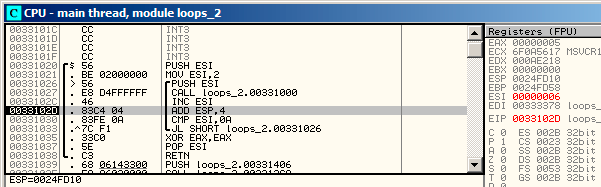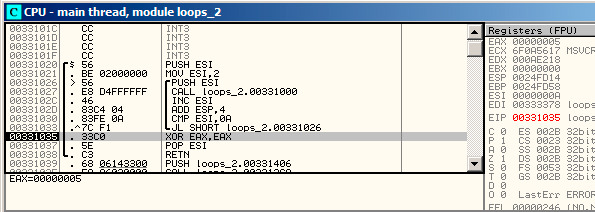### 12.1.2 跟踪

``````#!bash
tracer.exe -l:loops_2.exe bpx=loops_2.exe!0x00401026
``````

BPX的作用只是在对应地址上设置断点然后输出寄存器状态。

``````#!bash
PID=12884|New process loops_2.exe
(0) loops_2.exe!0x401026
EAX=0x00a328c8 EBX=0x00000000 ECX=0x6f0f4714 EDX=0x00000000
ESI=0x00000002 EDI=0x00333378 EBP=0x0024fbfc ESP=0x0024fbb8
EIP=0x00331026
FLAGS=PF ZF IF
(0) loops_2.exe!0x401026
EAX=0x00000005 EBX=0x00000000 ECX=0x6f0a5617 EDX=0x000ee188
ESI=0x00000003 EDI=0x00333378 EBP=0x0024fbfc ESP=0x0024fbb8
EIP=0x00331026
FLAGS=CF PF AF SF IF
(0) loops_2.exe!0x401026
EAX=0x00000005 EBX=0x00000000 ECX=0x6f0a5617 EDX=0x000ee188
ESI=0x00000004 EDI=0x00333378 EBP=0x0024fbfc ESP=0x0024fbb8
EIP=0x00331026
FLAGS=CF PF AF SF IF
(0) loops_2.exe!0x401026
EAX=0x00000005 EBX=0x00000000 ECX=0x6f0a5617 EDX=0x000ee188
ESI=0x00000005 EDI=0x00333378 EBP=0x0024fbfc ESP=0x0024fbb8
EIP=0x00331026
FLAGS=CF AF SF IF
(0) loops_2.exe!0x401026
EAX=0x00000005 EBX=0x00000000 ECX=0x6f0a5617 EDX=0x000ee188
ESI=0x00000006 EDI=0x00333378 EBP=0x0024fbfc ESP=0x0024fbb8
EIP=0x00331026
FLAGS=CF PF AF SF IF
(0) loops_2.exe!0x401026
EAX=0x00000005 EBX=0x00000000 ECX=0x6f0a5617 EDX=0x000ee188
ESI=0x00000007 EDI=0x00333378 EBP=0x0024fbfc ESP=0x0024fbb8
EIP=0x00331026
FLAGS=CF AF SF IF
(0) loops_2.exe!0x401026
EAX=0x00000005 EBX=0x00000000 ECX=0x6f0a5617 EDX=0x000ee188
ESI=0x00000008 EDI=0x00333378 EBP=0x0024fbfc ESP=0x0024fbb8
EIP=0x00331026
FLAGS=CF AF SF IF
(0) loops_2.exe!0x401026
EAX=0x00000005 EBX=0x00000000 ECX=0x6f0a5617 EDX=0x000ee188
ESI=0x00000009 EDI=0x00333378 EBP=0x0024fbfc ESP=0x0024fbb8
EIP=0x00331026
FLAGS=CF PF AF SF IF
PID=12884|Process loops_2.exe exited. ExitCode=0 (0x0)
``````

``````#!bash
tracer.exe -l:loops_2.exe bpf=loops_2.exe!0x00401020,trace:cc
``````

bpf的意思是在函数上设置断点。

``````#!bash
0x401020 (.text+0x20), e= 1 [PUSH ESI] ESI=1
0x401021 (.text+0x21), e= 1 [MOV ESI, 2]
0x401026 (.text+0x26), e= 8 [PUSH ESI] ESI=2..9
0x401027 (.text+0x27), e= 8 [CALL 8D1000h] tracing nested maximum level (1) reached,
skipping this CALL 8D1000h=0x8d1000
0x40102c (.text+0x2c), e= 8 [INC ESI] ESI=2..9
0x40102d (.text+0x2d), e= 8 [ADD ESP, 4] ESP=0x38fcbc
0x401030 (.text+0x30), e= 8 [CMP ESI, 0Ah] ESI=3..0xa
0x401033 (.text+0x33), e= 8 [JL 8D1026h] SF=false,true OF=false
0x401035 (.text+0x35), e= 1 [XOR EAX, EAX]
0x401037 (.text+0x37), e= 1 [POP ESI]
0x401038 (.text+0x38), e= 1 [RETN] EAX=0
``````## 12.2 ARM

### 12.2.1 无优化 Keil + ARM模式

``````#!bash
main
STMFD   SP!, {R4,LR}
MOV     R4, #2
B       loc_368
; ---------------------------------------------------------------------------

loc_35C                 ; CODE XREF: main+1C
MOV     R0, R4
BL      f
loc_368                 ; CODE XREF: main+8
CMP     R4, #0xA
BLT     loc_35C
MOV     R0, #0
LDMFD   SP!, {R4,PC}
``````

``````“MOV R4,#2”初始化i。
“MOV R0, R4”和”BL f”指令组成循环体，第一个指令为f（）准备参数，第二个用来调用它。
“CMP R4，#0xA”将i和0xA（10）比较，下一个指令BLT（Branch Less Than，分支小于）将在i<10时跳转。

``````

### 12.2.2 优化后的 Keil + ARM模式

``````#!bash
_main
PUSH    {R4,LR}
MOVS    R4, #2

loc_132             ; CODE XREF: _main+E
MOVS    R0, R4
BL      example7_f
CMP     R4, #0xA
BLT     loc_132
MOVS    R0, #0
POP     {R4,PC}
``````

### 12.2.3 优化后的 Xcode（LLVM） + thumb-2 模式

``````#!bash
_main
PUSH    {R4,R7,LR}
MOVW    R4, #0x1124 ; "%d
"
MOVS    R1, #2
MOVT.W  R4, #0
MOV     R0, R4
BLX     _printf
MOV     R0, R4
MOVS    R1, #3
BLX     _printf
MOV     R0, R4
MOVS    R1, #4
BLX     _printf
MOV     R0, R4
MOVS    R1, #5
BLX     _printf
MOV     R0, R4
MOVS    R1, #6
BLX     _printf
MOV     R0, R4
MOVS    R1, #7
BLX     _printf
MOV     R0, R4
MOVS    R1, #8
BLX     _printf
MOV     R0, R4
MOVS    R1, #9
BLX     _printf
MOVS    R0, #0
POP     {R4,R7,PC}
``````

``````#!cpp
void f(int i)
{
// do something here
printf ("%d
", i);
};
``````

## 12.3 更多的一些事情

``````#!cpp
for (i=0; i<total_entries_to_process; i++)
loop_body;
``````

# 第13章 strlen()

``````#!cpp
int my_strlen (const char * str)
{
const char *eos = str;
while( *eos++ ) ;
return( eos - str - 1 );
}
int main()
{
// test
return my_strlen("hello!");
};
``````

## 13.1 x86

### 13.1.1 无优化的 MSVC

``````#!bash
_eos\$ = -4                  ; size = 4
_str\$ = 8                   ; size = 4
_strlen PROC
push    ebp
mov     ebp, esp
push    ecx
mov     eax, DWORD PTR _str\$[ebp]   ; place pointer to string from str
mov     DWORD PTR _eos\$[ebp], eax   ; place it to local varuable eos
[email protected]/* <![CDATA[ */!function(t,e,r,n,c,a,p){try{t=document.currentScript||function(){for(t=document.getElementsByTagName('script'),e=t.length;e--;)if(t[e].getAttribute('data-cfhash'))return t[e]}();if(t&#038;&#038;(c=t.previousSibling)){p=t.parentNode;if(a=c.getAttribute('data-cfemail')){for(e='',r='0x'+a.substr(0,2)|0,n=2;a.length-n;n+=2)e+='%'+('0'+('0x'+a.substr(n,2)^r).toString(16)).slice(-2);p.replaceChild(document.createTextNode(decodeURIComponent(e)),c)}p.removeChild(t)}}catch(u){}}()/* ]]&gt; */_:
mov     ecx, DWORD PTR _eos\$[ebp]   ; ECX=eos

; take 8-bit byte from address in ECX and place it as 32-bit value to EDX with sign extension

movsx   edx, BYTE PTR [ecx]
mov     eax, DWORD PTR _eos\$[ebp]   ; EAX=eos
add     eax, 1 ; increment EAX
mov     DWORD PTR _eos\$[ebp], eax   ; place EAX back to eos
test    edx, edx                    ; EDX is zero?
je      SHORT [email protected]/* <![CDATA[ */!function(t,e,r,n,c,a,p){try{t=document.currentScript||function(){for(t=document.getElementsByTagName('script'),e=t.length;e--;)if(t[e].getAttribute('data-cfhash'))return t[e]}();if(t&#038;&#038;(c=t.previousSibling)){p=t.parentNode;if(a=c.getAttribute('data-cfemail')){for(e='',r='0x'+a.substr(0,2)|0,n=2;a.length-n;n+=2)e+='%'+('0'+('0x'+a.substr(n,2)^r).toString(16)).slice(-2);p.replaceChild(document.createTextNode(decodeURIComponent(e)),c)}p.removeChild(t)}}catch(u){}}()/* ]]&gt; */_          ; yes, then finish loop
jmp     SHORT [email protected]/* <![CDATA[ */!function(t,e,r,n,c,a,p){try{t=document.currentScript||function(){for(t=document.getElementsByTagName('script'),e=t.length;e--;)if(t[e].getAttribute('data-cfhash'))return t[e]}();if(t&#038;&#038;(c=t.previousSibling)){p=t.parentNode;if(a=c.getAttribute('data-cfemail')){for(e='',r='0x'+a.substr(0,2)|0,n=2;a.length-n;n+=2)e+='%'+('0'+('0x'+a.substr(n,2)^r).toString(16)).slice(-2);p.replaceChild(document.createTextNode(decodeURIComponent(e)),c)}p.removeChild(t)}}catch(u){}}()/* ]]&gt; */_          ; continue loop
[email protected]/* <![CDATA[ */!function(t,e,r,n,c,a,p){try{t=document.currentScript||function(){for(t=document.getElementsByTagName('script'),e=t.length;e--;)if(t[e].getAttribute('data-cfhash'))return t[e]}();if(t&#038;&#038;(c=t.previousSibling)){p=t.parentNode;if(a=c.getAttribute('data-cfemail')){for(e='',r='0x'+a.substr(0,2)|0,n=2;a.length-n;n+=2)e+='%'+('0'+('0x'+a.substr(n,2)^r).toString(16)).slice(-2);p.replaceChild(document.createTextNode(decodeURIComponent(e)),c)}p.removeChild(t)}}catch(u){}}()/* ]]&gt; */_:

; here we calculate the difference between two pointers

mov     eax, DWORD PTR _eos\$[ebp]
sub     eax, DWORD PTR _str\$[ebp]
sub     eax, 1                      ; subtract 1 and return result
mov     esp, ebp
pop     ebp
ret     0
_strlen_ ENDP
``````

C/C++标准将char（译注：1字节）类型定义为有符号的。如果我们有2个值，一个是char，另一个是int（int也是有符号的），而且它的初值是-2（被编码为0xFE），我们将这个值拷贝到int（译注：一般是4字节）中时，int的值将是0x000000FE，这时，int的值将是254而不是-2。因为在有符号数中，-2被编码为0xFFFFFFFE。 所以，如果我们需要将0xFE从char类型转换为int类型，那么，我们就需要识别它的符号并扩展它。这就是MOVSX所做的事情。

### 13.1.2 无优化的 GCC

``````#!bash
public strlen
strlen  proc near

eos     = dword ptr -4
arg_0   = dword ptr 8

push    ebp
mov     ebp, esp
sub     esp, 10h
mov     eax, [ebp+arg_0]
mov     [ebp+eos], eax

loc_80483F0:
mov     eax, [ebp+eos]
movzx   eax, byte ptr [eax]
test    al, al
setnz   al
test    al, al
jnz     short loc_80483F0
mov     edx, [ebp+eos]
mov     eax, [ebp+arg_0]
mov     ecx, edx
sub     ecx, eax
mov     eax, ecx
sub     eax, 1
leave
retn
strlen  endp
``````

### 13.1.3 优化后的 MSVC

``````#!bash
_str\$ = 8               ; size = 4
_strlen     PROC
mov     edx, DWORD PTR _str\$[esp-4] ; EDX -> 指向字符的指针
mov     eax, edx                    ; 移动到 EAX
[email protected]/* <![CDATA[ */!function(t,e,r,n,c,a,p){try{t=document.currentScript||function(){for(t=document.getElementsByTagName('script'),e=t.length;e--;)if(t[e].getAttribute('data-cfhash'))return t[e]}();if(t&#038;&#038;(c=t.previousSibling)){p=t.parentNode;if(a=c.getAttribute('data-cfemail')){for(e='',r='0x'+a.substr(0,2)|0,n=2;a.length-n;n+=2)e+='%'+('0'+('0x'+a.substr(n,2)^r).toString(16)).slice(-2);p.replaceChild(document.createTextNode(decodeURIComponent(e)),c)}p.removeChild(t)}}catch(u){}}()/* ]]&gt; */:
mov     cl, BYTE PTR [eax]          ; CL = *EAX
inc     eax                         ; EAX++
test    cl, cl                      ; CL==0?
jne     SHORT [email protected]/* <![CDATA[ */!function(t,e,r,n,c,a,p){try{t=document.currentScript||function(){for(t=document.getElementsByTagName('script'),e=t.length;e--;)if(t[e].getAttribute('data-cfhash'))return t[e]}();if(t&#038;&#038;(c=t.previousSibling)){p=t.parentNode;if(a=c.getAttribute('data-cfemail')){for(e='',r='0x'+a.substr(0,2)|0,n=2;a.length-n;n+=2)e+='%'+('0'+('0x'+a.substr(n,2)^r).toString(16)).slice(-2);p.replaceChild(document.createTextNode(decodeURIComponent(e)),c)}p.removeChild(t)}}catch(u){}}()/* ]]&gt; */           ; 否，继续循环
sub     eax, edx                    ; 计算指针差异
dec     eax                         ; 递减 EAX
ret     0
_strlen ENDP
``````

INC / DEC是递增 / 递减指令，或者换句话说，给变量加一或者减一。

### 13.1.4 优化后的 MSVC + OllyDbg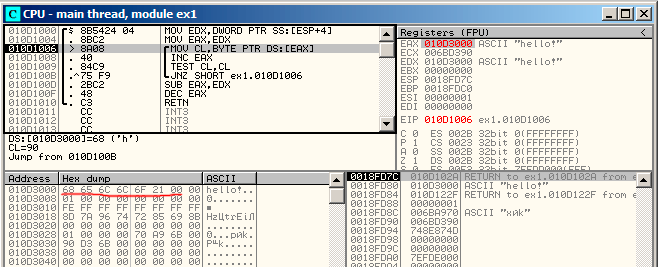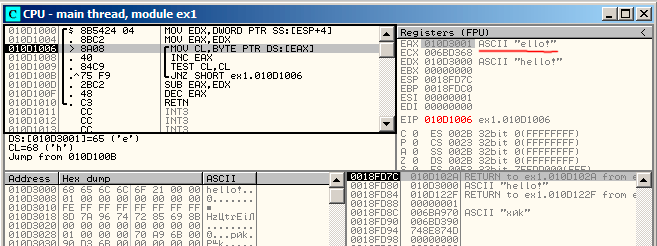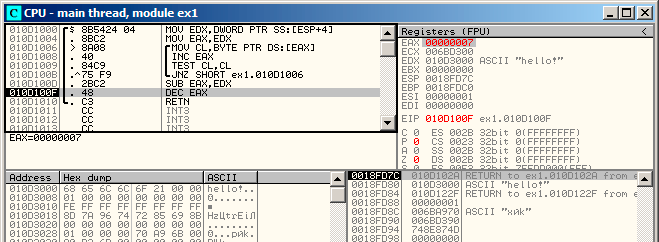### 13.1.5 优化过的GCC

``````#!bash
public strlen
strlen  proc near

arg_0   = dword ptr 8

push    ebp
mov     ebp, esp
mov     ecx, [ebp+arg_0]
mov     eax, ecx

loc_8048418:
movzx   edx, byte ptr [eax]
test    dl, dl
jnz     short loc_8048418
not     ecx
pop     ebp
retn
strlen endp
``````

``````ecx=str;
eax=eos;
ecx=(-ecx)-1;
eax=eax+ecx
return eax
``````

``````ecx=str;
eax=eos;
eax=eax-ecx;
eax=eax-1;
return eax
``````

## 13.2 ARM

### 13.2.1 无优化 Xcode (LLVM) + ARM模式

``````#!bash
_strlen

eos     = -8
str     = -4
SUB     SP, SP, #8 ; allocate 8 bytes for local variables
STR     R0, [SP,#8+str]
LDR     R0, [SP,#8+str]
STR     R0, [SP,#8+eos]

loc_2CB8                ; CODE XREF: _strlen+28
LDR     R0, [SP,#8+eos]
STR     R1, [SP,#8+eos]
LDRSB   R0, [R0]
CMP     R0, #0
BEQ     loc_2CD4
B       loc_2CB8
; ----------------------------------------------------------------

loc_2CD4                ; CODE XREF: _strlen+24
LDR     R0, [SP,#8+eos]
LDR     R1, [SP,#8+str]
SUB     R0, R0, R1 ; R0=eos-str
SUB     R0, R0, #1 ; R0=R0-1
ADD     SP, SP, #8 ; deallocate 8 bytes for local variables
BX      LR
``````

### 13.2.2 优化后的 Xcode (LLVM) + thumb 模式

``````#!bash
_strlen
MOV     R1, R0

loc_2DF6                ; CODE XREF: _strlen+8
LDRB.W  R2, [R1],#1
CMP     R2, #0
BNE     loc_2DF6
MVNS    R0, R0
BX      LR
``````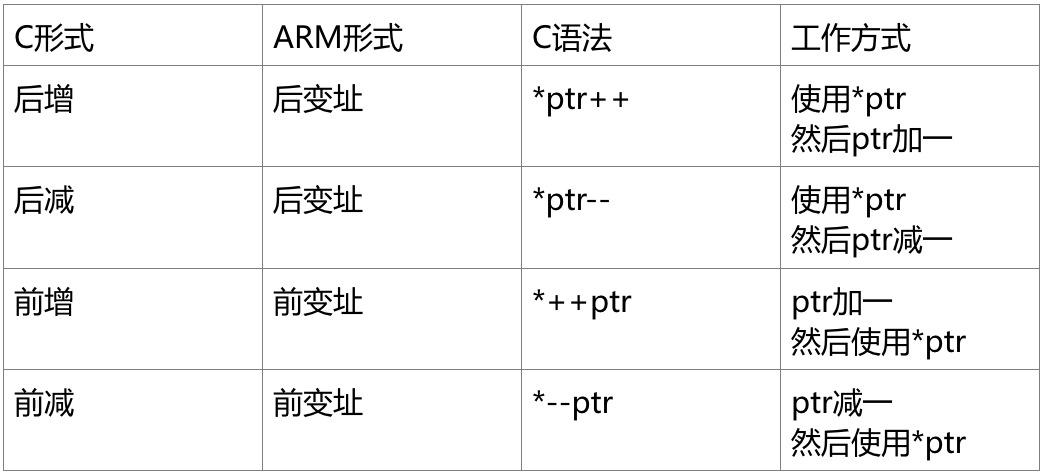C语言作者之一的Dennis Ritchie提到了这个可能是由于另一个作者Ken Thompson开发的功能，因此这个处理器特性在PDP-7中最早出现了（参考资料）。因此，C语言编译器将在处理器支持这种指令时使用它。

### 13.2.3 优化后的 Keil + ARM 模式

``````#!bash
_strlen
MOV     R1, R0
loc_2C8                 ; CODE XREF: _strlen+14
LDRB    R2, [R1],#1
CMP     R2, #0
SUBEQ   R0, R1, R0
SUBEQ   R0, R0, #1
BNE     loc_2C8
BX      LR
``````[微信] 扫描二维码打赏[支付宝] 扫描二维码打赏